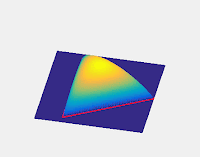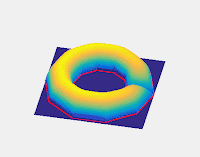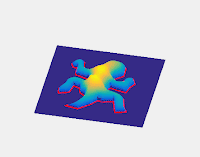## A Circle Theorem

Take a circle of radius $R$. Select a point $A$ inside it a distance $a$ from the center, with $a < R$. From $A$, construct $N>1$ line segments starting from A and touching the circle, segment k touching the circle at $P_k$, such that if $a-b\equiv \pm 1 \mod N$, then $\measuredangle P_aAP_b=2\pi/N$, that is all the segments are equally-angularly-spaced. Let $d_k=\overline{AP_k}$. Then $\prod_{k=1}^{N}d_k=\prod_{k=1}^{N}\left ( a\cos \left ( \theta_0+\frac{2 k \pi}{N} \right )+\sqrt{R^2-a^2+a^2\cos^2 \left ( \theta_0+\frac{2 k \pi}{N} \right )} \right ) \\ \prod_{k=1}^{N}d_k=\prod_{k=1}^{N}\left (\sqrt{R^2-a^2}\exp\left ( \sinh^{-1}\left (\frac{a}{\sqrt{R^2-a^2}}\sin \left ( \theta'_0+\frac{2 k \pi}{N} \right ) \right ) \right ) \right )$ Therefore $\sqrt[N]{\prod_{k=1}^{N}d_k}=\sqrt{R^2-a^2}\exp \left (\frac{1}{N}\sum_{k=1}^{N} \sinh^{-1}\left (\frac{a}{\sqrt{R^2-a^2}}\sin \left ( \theta'_0+\frac{2 k \pi}{N} \right ) \right ) \right )$ It follows that, for N even, as the summation will cancel in every term, $\sqrt[N]{\prod_{k=1}^{N}d_k}=\sqrt{R^2-a^2}$ This also holds asymptotically, as the error approaches zero. it is generally not true for N odd.

Note that this much more widely generalizes the well-known geometric mean theorem. This can be seen as a consequence of the power of a point theorem.

A pleasant interpretation of this is that if we take a diametric cross-section of a sphere and choose a point on that disk, the height of the sphere above that point is the geometric mean of the legs of any $2N>1$ equiangular, planar, stellar net connecting that point to the boundary of the disk.

## A Related Volumizing Algorithm

This theorem suggests an algorithm for producing a 3D volume given a closed 2D boundary shape. If we assume the 2D shape is of a diametric cross-section, we simply apply the method detailed above to produce the height above that point. That is, for a given point inside the shape, we take an N-leg equiangular stellar net emanating from that point to the boundary of the shape. The height of the surface at that point is then the geometric mean of the N legs of that net.

This method ensures that circular shapes produce spherical surfaces. However, if N is low, for less regular boundary shapes, the resulting surface may be quite lumpy or sensitive to how the angles of each net are chosen. One solution, then, is simply to make N large enough. However, this may end up being computationally expensive.

In theory, it may be possible to find the asymptotic value: find all parts of the boundary shape visible from the given point, and find the integral of the log of the distance, sweeping over the angle. If the boundary is a polygon, this involves evaluating (or approximating) integrals of the form $\int\ln\left ( \sin(x) \right)dx$ Which have no general closed form in terms of elementary functions. However, we can evaluate certain cases. One easy example is that of an infinite corridor formed from two parallel lines. We find that the height profile is double that of a circular cylinder. It may be desirable, then, to determine another function to multiply by which will halve the heights of corridors but leave hemispheres undisturbed.

Below we give some visual examples of the results of the algorithm. The original 2D shapes are shown in red.In order, an equilateral triangle, an icosagon, a five-pointed star, an almost-donut, an Escherian tesselating lizard, and a tesselating spider.

#### 1 comment:

1.If would possibly be} in need of professional sandblasting, contact Tymetal. We have the experience and gear to deal with a variety of blasting methods. Sand Blasting – best fins for freediving The process of blasting surfaces and removing unwanted coatings and coverings together with rust, oxidization, paints, and more.